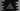# C program to swap two bits of a number## C program to swap two bits of a number:

In this C program, we will learn how to swap two bits of a given number. This program will take the number and bits as input from the user, swap them and print the output in decimal.

With this program, you will learn how to take user inputs in C and how to do bit manipulation.

### Algorithm:

The idea is to use XOR. XOR of two bits gives 1 only if both bits are different. Else, it gives 0.

For example, for 57, its binary value is:

``111001``

Let’s try to swap the 0th and 2nd bit,

• 0th bit is 1
• 2nd bit is 0

The XOR of these bits are 1 XOR 0 i.e. 1. Now, if we do XOR of 1 with the 0th and 2nd bits, it will swap both bits.

``````111001
XOR
000101
------
111100``````

So, 111100 is the binary value of the required number once we swapped the 0th and 2nd bits.

## C program:

Below is the complete C program:

``````#include <stdio.h>

int swapBitsNumber(unsigned int num, unsigned int firstPosition, unsigned int secondPosition)
{
unsigned int firstBit = (num >> firstPosition) & 1;

unsigned int secondBit = (num >> secondPosition) & 1;

unsigned int xorBit = (firstBit ^ secondBit);

xorBit = (xorBit << firstPosition) | (xorBit << secondPosition);

return num ^ xorBit;
}

int main()
{
int number, firstPosition, secondPosition, result;

printf("Enter a number: ");
scanf("%d", &number);

printf("Enter the first bit position: ");
scanf("%d", &firstPosition);

printf("Enter the second bit position: ");
scanf("%d", &secondPosition);

result = swapBitsNumber(number, firstPosition, secondPosition);
printf("Number after bits are swapped: %d\n", result);

return 0;
}``````

Here,

• swapBitsNumber method is used to swap two bits of a number. It takes the number, first position and second position of the bits as the parameters and returns the new number by swapping the bits.
• firstBit is the first bit of the number at firstPosition and secondBit is the second bit of the number at secondPosition.
• xorBit is the value calculated by using XOR of firstBit and secondBit.
• We are then moving the xorBit to firstPosition and secondPosition with all remaining positions as 0.
• The last line is using XOR with the number and xorBit to find the final value.

### Output:

If you run the above program, it will print output as like below:

``````Enter a number: 58
Enter the first bit position: 0
Enter the second bit position: 2
Number after bits are swapped: 58

Enter a number: 58
Enter the first bit position: 0
Enter the second bit position: 3
Number after bits are swapped: 51``````

58 is : 111010. If we swap the bits at position 0 and 2, it will be 111010 i.e. 58. If we swap the bits at position 0 and 3, it will be 110011 i.e. 51.